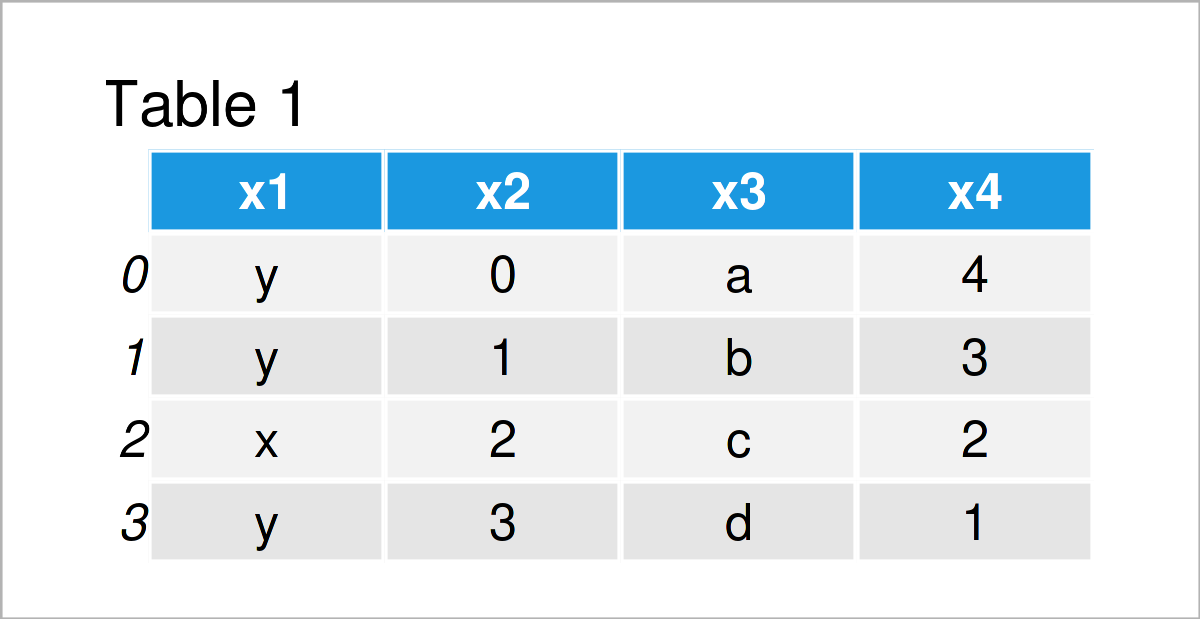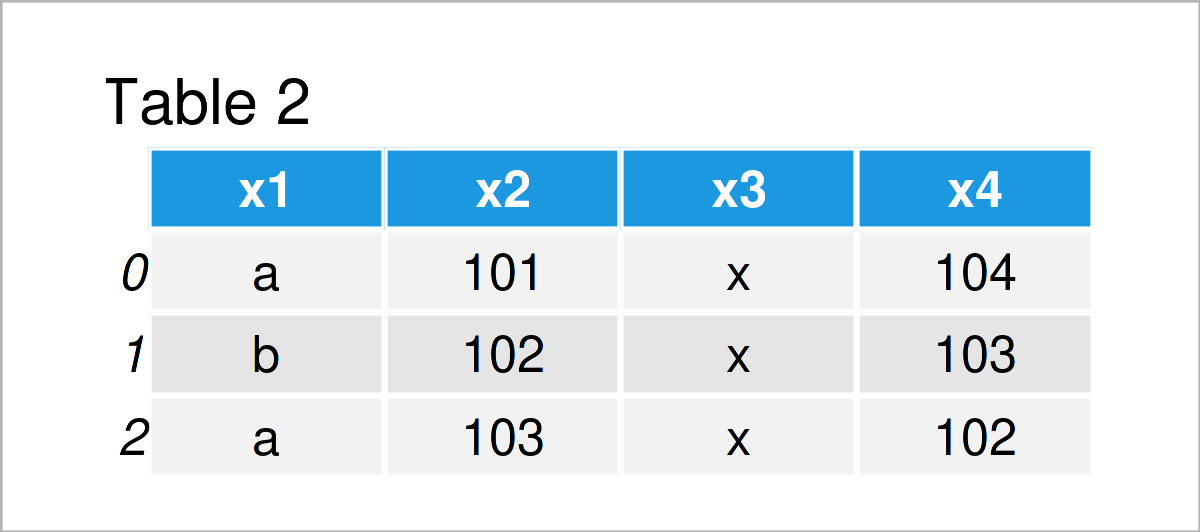# Append Multiple pandas DataFrames in Python (Example)

In this Python tutorial you’ll learn how to concatenate three or more pandas DataFrames.

The article will consist of one example for the concatenation of three or more pandas DataFrames. More precisely, the post consists of this:

Let’s do this:

## Example Data & Libraries

To be able to use the functions of the pandas library, we first need to import pandas:

`import pandas as pd                                              # Import pandas library`

We also have to construct several example DataFrames that we can use in the following examples:

```data1 = pd.DataFrame({"x1":["y", "y", "x", "y"],                 # Create first pandas DataFrame
"x2":range(0, 4),
"x3":["a", "b", "c", "d"],
"x4":range(4, 0, - 1)})
print(data1)                                                     # Print first pandas DataFrame``````data2 = pd.DataFrame({"x1":["a", "b", "a"],                      # Create second pandas DataFrame
"x2":range(101, 104),
"x3":["x", "x", "x"],
"x4":range(104, 101, - 1)})
print(data2)                                                     # Print second pandas DataFrame``````data3 = pd.DataFrame({"x1":["z", "zz", "zzz", "zzzz", "zzzzz"],  # Create third pandas DataFrame
"x2":range(10, 15),
"x3":["xxxxx", "xxxx", "xxxx", "xx", "x"],
"x4":range(15, 10, - 1)})
print(data3)                                                     # Print third pandas DataFrame```As you can see based on the previous tables, we have created three different pandas DataFrames called data1, data2, and data3.

Each of our data sets comprises the four columns x1, x2, x3, and x4. However, the values within those DataFrames are different compared to each other.

Let’s stack these pandas DataFrames into a single DataFrame!

## Example: Combine Three pandas DataFrames Using concat() Function

This example illustrates how to append multiple pandas DataFrames on top of each other.

For this task, we can apply the concat function as shown in the following Python code:

```data_all3 = pd.concat([data1, data2, data3],                     # Append three pandas DataFrames
ignore_index = True,
sort = False)
print(data_all3)                                                 # Print combined DataFrame```Table 4 shows the output of the previous Python code: We have created a new pandas DataFrame, which is a union of our 3 input DataFrames.

## Video & Further Resources

Do you need more explanations on how to add multiple pandas DataFrame on top of each other?

Then you might want to watch the following video on the Statistics Globe YouTube channel. In the video instruction, I’m illustrating the Python programming code of this post:

Please accept YouTube cookies to play this video. By accepting you will be accessing content from YouTube, a service provided by an external third party.If you accept this notice, your choice will be saved and the page will refresh.

In addition, you may want to read the related articles on my website. You can find a selection of tutorials below:

In summary: In this tutorial, I have shown how to join, merge, and rbind three or more pandas DataFrames in Python. Please let me know in the comments section, if you have further questions.

Subscribe to the Statistics Globe Newsletter